Home > CC4 > Chapter 11 > Lesson 11.1.3 > Problem11-34

11-34.
1. Solve the following inequalities for x. Graph your solutions on a number line. Homework Help ✎

2.  a. 3x − 5 ≤ 7 + 2x b.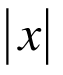−3 < 7 c. 5(2 − x) + 6 > 16 d.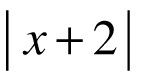> 3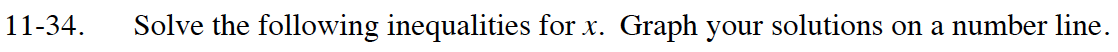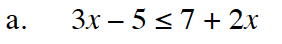Treat the inequality like an equation, then solve to find the boundary point.

Graph the point on a number line then test another point to see which way to shade.

x ≤ 12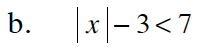Solve for the absolute value of x, then make two equations.

Test numbers to determine which region is the solution.

− 10 < x < 10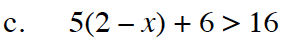Distribute, then solve for x.

x < 0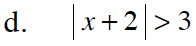Solve x + 2 = 3 and x + 2 = −3 to find the boundary points.